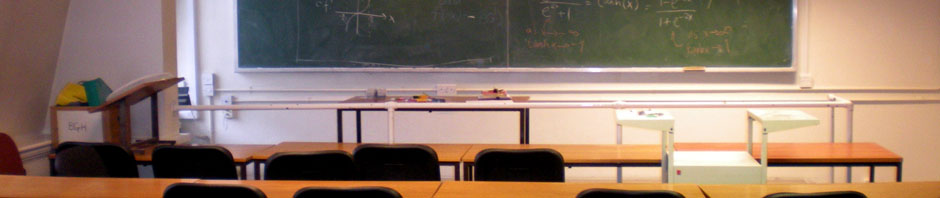# Mathematical Methods for Arts and SciencesThis page corresponds to the UCL first year mathematics-for-BASc course MATH1403, Mathematical Methods for Arts and Sciences, which I had the pleasure of teaching in the autumns of 2012, 2013 and 2014.

Since 2015, the course has been renumbered MATH0012 and is taught by the amazing Dr Luciano Rila.

### Course aims and objectives

The aim of this course is to bring students from a background of diverse A-level (and similar) syllabuses to a uniform level of confidence and competence in basic calculus, a subject which is of basic importance not only in most areas of mathematics, but also in science in general.

It is designed for BASc students, and covers topics with an eye kept on both the other first-year modules and the options available further down students’ chosen pathways.

The course covers complex numbers, standard functions of a real variable, methods of integration and an introduction to ordinary differential equations, as well as introductions to partial differentiation and Fourier series.

Each topic is given a formal treatment and illustrated by examples of varying degrees of difficulty. It is intended that the approach to the subject matter should also stimulate those with an extensive A-level background.

It is a demanding course, intending to bring students to a level where they are able to join courses offered by departments in the MAPS faculty with the rest of that department’s cohort.

### Syllabus

As it stood when I taught it, the course content was:

1. Differentiation: from first principles, revision of material from A-level, hyperbolic functions, partial differentiation, Taylor series.
2. Integration: from first principles, partial fractions, integration by substitution, integration by parts, integration by reduction formulae, improper integrals.
3. Complex numbers: history and motivation, Argand diagram, exponential representation (feat. Euler’s identity), roots of complex numbers, complex logarithms.
4. Differential equations: linear and nonlinear first order ordinary differential equations, linear second order ordinary differential equations with constant coefficients (complementary function and particular integral).
5. Fourier series: history and motivation, periodic functions, formula for coefficients.